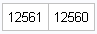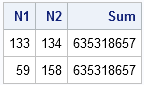John D. Cook posted a story about Hardy, Ramanujan, and Euler and discusses a conjecture in number theory from 1937. Cook says,

Euler discovered 635,318,657 = 158^4 + 59^4 = 134^4 + 133^4 and that this was the smallest [integer]known to be the sum of two fourth powers in two ways. It seems odd now to think of such questions being unresolved. Today we’d ask Hardy “What do you mean 635318657 is the smallest known example? Why didn’t you write a little program to find out whether it really is the smallest?”

Cook goes on to encourage his readers to write a program that settles the conjecture, which is obviously no longer an open problem. Here's a SAS/IML solution. First, introduce a function that I use a lot:

```proc iml; start ExpandGrid( x, y ); /* Return ordered pairs on a uniform grid of points */ Nx = nrow(x); Ny = nrow(y); x = repeat(x, Ny); y = shape( repeat(y, 1, Nx), 0, 1 ); return ( x || y ); finish;```

The ExpandGrid function generates a uniform grid of all ordered pairs of it's arguments. To solve the "conjecture," generate all ordered pairs that contain the integers 1–158 and check to see if the sum of fourth powers contain duplicate values. The number 158 is the greatest integer that is less than the fourth root of 635,318,657.

```/* settle conjecture of Euler/Hardy: Is 635,318,657 the smallest integer that can be written as the sum of 4th powers in two ways? */ k = floor(635318657##0.25); /* only need to test up to 4th root */ g = ExpandGrid(t(1:k), t(1:k)); /* all ordered pairs */ g = g[ loc(g[,1] <= g[,2]), ]; /* omit symmetric pairs */ f = g[,1]##4 + g[,2]##4; /* sum of 4th powers */ print (nrow(f)) (ncol(unique(f))); /* exactly one sum is the same */```The output proves the conjecture: there are 12,561 ordered pairs of integers to consider, and there are 12,560 unique sums of fourth powers. That means that there is exactly one sum of fourth powers that can be written in two different ways, and Euler has already told us the particular values.

If you didn't know Euler's results, you could use the same computation to find Euler's values: sort the numbers by the sum of fourth powers and use the DIF function to take differences between consecutive elements. Print out the rows for which the difference is zero (that is, that the sum of fourth powers are equal):

```/* find the actual pairs of integers that have the same sum */ g = g || f; /* concatenate fourth powers onto pairs */ call sort(g, 3); /* sort by sum of 4th powers */ idx = loc(dif(g[,3])=0); /* dif=0 <==> consecutive values equal */ print (g[idx-1:idx, ])[c={N1 N2 "Sum"}];```It is fun to think of what someone like Euler might have accomplished if had the tools that we do today!

ShareDistinguished Researcher in Computational Statistics

Rick Wicklin, PhD, is a distinguished researcher in computational statistics at SAS and is a principal developer of SAS/IML software. His areas of expertise include computational statistics, simulation, statistical graphics, and modern methods in statistical data analysis. Rick is author of the books Statistical Programming with SAS/IML Software and Simulating Data with SAS.

1.Fermat's Last Theorem - Go! :D

•Fortunately for the mathematicians, computers still have a hard time with theorems that require checking infinitely many possibilities!

2.For those without IML, something similar to Rick's program can be achieved using PROC SQL. Try the code below to generate the first 16 numbers of this type. Or replace 1000 with 9600 to extend the search to as far as an integer can be exactly represented under Windows. While n=1000 takes less than 1 second, n=9600 takes about 1 min on my machine.

```data n; do i=1 to 1000; output; end; run; ; proc sql; create table t as select a.i as i1, b.i as i2, i1**4 + i2**4 as s from n a, n b where i1 < i2 order by s; quit; ; data _null_; set t; i3=lag(i1); i4=lag(i2); if s=lag(s) then put (i3 i4 i1 i2) (5.0) s comma22.0; run;```

```**************** OUTPUT ******************
59  158  133  134           635,318,657
7  239  157  227         3,262,811,042
256  257  193  292         8,657,437,697
266  268  118  316        10,165,098,512
399  402  177  474        51,460,811,217
14  478  314  454        52,204,976,672
271  502  298  497        68,899,596,497
359  514  103  542        86,409,838,577
512  514  386  584       138,519,003,152
222  631  503  558       160,961,094,577
532  536  236  632       162,641,576,192
21  717  471  681       264,287,694,402
665  670  295  790       397,074,160,625
768  771  579  876       701,252,453,457
354  948  798  804       823,372,979,472
28  956  628  908       835,279,626,752
```